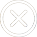400-1888-810

# rsi参数设置多少好？股票rsi指标三线参数设置？终于弄清楚了！

354次　 2023-03-14　分类：证券文章

LC:=REF(CLOSE,1);

RSI1:SMA(MAX(CLOSE-LC,0),5,1)/SMA(ABS(CLOSE-LC),5,1)*100;

RSI2:SMA(MAX(CLOSE-LC,0),10,1)/SMA(ABS(CLOSE-LC),10,1)*100;

RSI3:SMA(MAX(CLOSE-LC,0),14,1)/SMA(ABS(CLOSE-LC),14,1)*100;

A1:=RSI1>80 AND RSI2>80 AND RSI3>80;

A2:=RSI1<20 AND RSI2<20 AND RSI3<20;

DRAWTEXT(A1,RSI1-2,'快见顶了');

DRAWTEXT(A2,RSI1+2,'快见底了');

A3:=A2 AND CROSS(RSI1,RSI3);

DRAWTEXT(A3,RSI1+2,'超级买点') COLORYELLOW;

A4:=A1 AND CROSS(RSI3,RSI1);

DRAWTEXT(A4,RSI1+2,'超级卖点') COLORYELLOW;

C3:=BARSLAST(REF(CROSS("RSI.RSI2","RSI.RSI1"),1));

D3:=REF(C,C3+1) "RSI.RSI1" AND CROSS("RSI.RSI2","RSI.RSI1");

DRAWTEXT(D3> 0,RSI1-2,'RSI顶背离'),COLORFF9900;(RSI顶背离;)

AA3:=BARSLAST(REF(CROSS("RSI.RSI1","RSI.RSI2"),1));

B3:=REF(C,AA3+1)> C AND REF("RSI.RSI1",AA3+1)<"RSI.RSI1" AND CROSS("RSI.RSI1","RSI.RSI2");

DRAWTEXT(B3> 0,RSI1+2,'RSI底背离'),COLOR0000FF;(RSI底背离;)## 证券问题标签

dr股票

xd是什么意思

st股票

KDJ